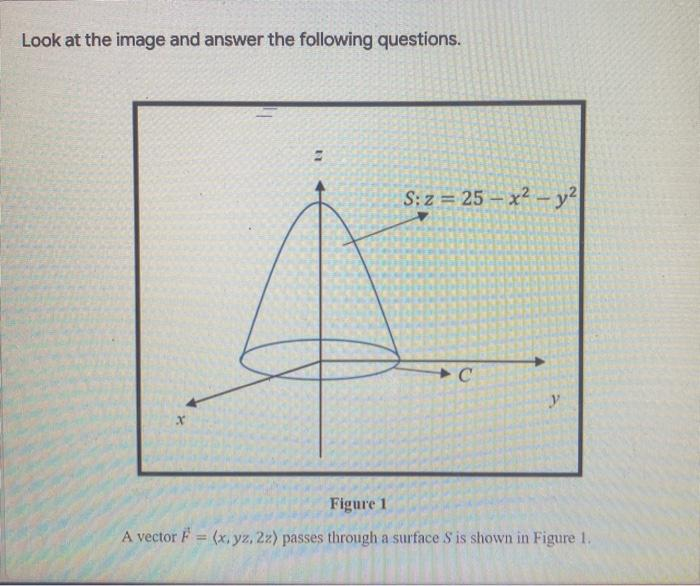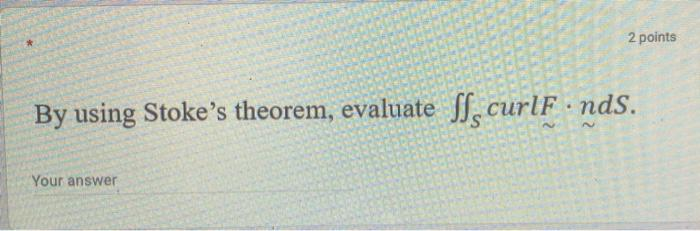### Create an Account

Already have account?

### Forgot Your Password ?

Home / Questions / Look at the image and answer the following questions. S: z = 25 – x2 - y2 с y Figure 1 A v...

# Look at the image and answer the following questions. S: z = 25 – x2 - y2 с y Figure 1 A vector F = (x, yz, 22) passes through a surface S is shown in Figure 1. 2 points By using Stoke's theorem, eval

Look at the image and answer the following questions. S: z = 25 – x2 - y2 с y Figure 1 A vector F = (x, yz, 22) passes through a surface S is shown in Figure 1.
2 points By using Stoke's theorem, evaluate SS curlF.nds. Your answerApr 14 2021 View more View Less

#### Answer (Solved)Subscribe To Get Solution